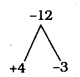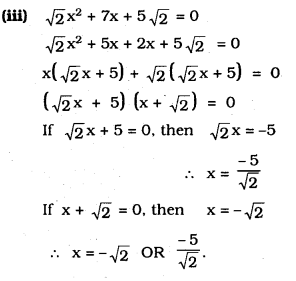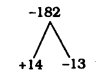# KSEEB SSLC Class 10 Maths Solutions Chapter 10 Quadratic Equations Ex 10.2

KSEEB SSLC Class 10 Maths Solutions Chapter 10 Quadratic Equations Ex 10.2 are part of KSEEB SSLC Class 10 Maths Solutions. Here we have given Karnataka SSLC Class 10 Maths Solutions Chapter 10 Quadratic Equations Exercise 10.2.

## Karnataka SSLC Class 10 Maths Solutions Chapter 10 Quadratic Equations Exercise 10.2

Question 1.
Find the roots of the following quadratic equations by factorisation:
(i) x2 – 3x – 10 = 0 ,
(ii) 2x2 + x – 6 = 0
(iii) $$\sqrt{2}$$x2 + 7x + $$5 \sqrt{2}$$ =0
(iv) 2x2 – x + $$\frac{1}{8}$$ = 0
(v) 100x2 – 20x + 1 = 0
Solution:
(i) x2 – 3x – 10 = 0
x2 – 5x + 2x – 10 = 0x(x – 5) + 2 (x – 5) = 0
(x – 5) (x + 2) = 0
If x + 2 = 0, then x = -2
If x – 5 = 0, then x = 5
∴ x = -2 OR +5.

(ii) 2x2 + x – 6 = 0
2x2 + 4x – 3x – 6 = 02x(x + 2) – 3(x + 2) = 0
(x + 2) (2x – 3) = 0
If x + 2 = 0, then x = -2
If 2x – 3 = 0, then 2x = 3 x = $$\frac{3}{2}$$
∴ x = -2 OR $$\frac{3}{2}$$iv) 2x2 – x + $$\frac{1}{8}$$ = 0 Multiply by 8
8(2x2 -x + $$\frac{1}{8}$$) = 0
16x2 – 8x + $$\frac{8}{8}$$ = 0
16x2 – 8x + 1 = 0
16x2 – 4x – 4x + 1 = 0
4x (4x – 1) – 1 (4x – 1) =
(4x – 1) (4x – 1) = 0
4x – 1 = 0 or 4x – 1 = 0
4x = 1 or 4x = 1
x = $$\frac{1}{4}$$ or x = $$\frac{1}{4}$$
x = $$\frac{1}{4}$$ are the roots of the equation 2x2 – x + $$\frac{1}{8}$$ = 0

(v) 100x2 – 20x + 1 = 0
100x2 – 10x – 10x + 1 = 0
10x(10x – 1) – 1 (10x – 1) = 0
(10x – 1) (10x – 1) = 0
(10x – 1)2 = 0
If 10x – 1 = 0, then
10x = 1
∴ x = $$\frac{1}{10}$$
∴ Two roots are $$\frac{1}{10} , \frac{1}{10}$$Question 2.
Solve the problems:
(i) John and Jivanti together have 45 marbles. Both of them lost 5 marbles each, and the product of the number of marbles they now have is 124. We would like to find out how many marbles they had to start with.
(ii) A cottage industry produces a certain number of toys in a day. The cost of production of each toy (in rupees) was found to be 55 minus the number of toys produced in a day. On a particular day, the total cost of production was ₹ 750. We would like to find out the number of toys produced on that day.
Solution:
(i) Let John had x marbles and Jivanti had (45 – x) marbles.
When both of them lost 5 marbles then equation becomes (x – 5) × (45 – x – 5) = 124
⇒ (x – 5) × (40 – x) = 124
⇒ x2 – 45x + 324 = 0
⇒ x2 – 9x – 36x + 324 = 0
⇒ x(x – 9) – 36(x – 9) = 0
⇒ (x – 9)(x – 36) = 0
Either x – 9 = 0 or x – 36 = 0
Thus, x = 9 or x = 36
∴ If John had 9 marbles, then Jivanti had 45 – 9 = 36 marbles.
If John had 36 marbles, then Jivanti had 45 – 36 = 9 marbles.

(ii) Let the number of toys produced in a day be x.
Then cost of 1 toy = $$\frac{750}{x}$$
⇒ $$\frac{750}{x}$$ = 55 – x
⇒ 750 = 55x – x2
⇒ x2 – 55x + 750 = 0
⇒ x2 – 30x – 25x + 750 = 0
⇒ x(x – 30) – 25(x – 30) = 0
⇒ (x – 30)(x – 25) = 0
Either x – 30 = 0 or x – 25 = 0
x = 30 or x = 25Question 3.
Find two numbers whose sum is 27 and the product is 182.
Solution:
Sum of two numbers be 27
Let one number be ‘x’ other number be ’27 – x’
Product of two numbers = 182
x(27 – x)= 182
27x – x2 = 182
x2 – 27x + 182 = 0
x2 – 14x – 13x+ 182 = 0
x (x – 14) – 13 (x – 14) = 0
(x – 14) (x – 13) = 0
x + 14 = 0 (or) x – 13 = 0
x = – 14 or x = 13
x = 14, 13
∴ Two numbers are 14, 13 (or) 13, 14

Question 4.
Find two consecutive positive integers, sum of whose squares is 365.
Solution:
In that numbers, let one of the numbers be ’x’.
It’s consecutive positive integer is (x + 1)
Sum of their squares is 365.
∴ (x)2 + (x + 1)2 = 365
x2 + x2 + 2x + 1 = 365
2x2 + 2x + 1 = 365
2x2 + 2x + 1 – 365 = 0
2x2 + 2x – 365 = 0
x2 + x – 182 = 0x2 + 14x – 13x – 182 = 0
x(x + 14) – 13(x + 14) = 0
(x + 14) (x – 13) = 0
If x + 14 = 0, then x = -14
If x – 13 = 0, then x = 13
In these positive integer is 13.
∴ One number, x = 13
It consecutive number is, x + 1
= 13 + 1 = 14
∴ The Numbers are 14 and 13.Question 5.
The altitude of a right triangle is 7 cm less than its base. If the hypotenuse is 13 cm., find the other two sides.
Solution:In ⊥∆ABC,
∠ABC = 90°.
AB is altitude,
BC is base AC is Hypotenuse
Let Base, BC = x cm.
if so considered, :
Altitude AB = (x – 7) cm.
Hypotenuse AC = 13 cm.
As per Pythagoras theorem,
In a right angled ABC,
AB2 + BC2 = AC2
(x – 7)2 + (x)2 = (13)2
x2 – 14x + 49 + x2= 169
2x2 – 14x + 49 = 169
2x2 – 14x + 49 – 169 = 0
2x2 – 14x – 120 = 0
x2 – 7x – 60 = 0x2 – 12x + 5x – 6 0 = 0
x(x – 12) + 5(x – 12) = o
(x – 12) (x + 5) =0
If x – 12 = 0, then x = 12
If x + 5 = 0, then x = -5
Positive value, x = 12
∴ Base, BC = x = 12 cm.
Altitude, AB = x – 7 = 12 – 7 = 5 cm.Question 6.
A cottage industry produces a certain number of pottery articles in a day. It was observed on a particular day that the cost of production of each article (in rupees) was 3 more than twice the number of articles produced on that day. If the total cost of production on that day was ₹ 90, find the number of articles produced and the cost of each article.
Solution:
Let the number of articles produced in a day = x
∴ Cost of production of each article = ₹ (2x + 3)
According to the condition,
Total cost = ₹ 90
⇒ x × (2x + 3) = 90
⇒ 2x2 + 3x = 90
⇒ 2x2 + 3x – 90 = 0
⇒ 2x2 – 12x + 15x – 90 = 0
⇒ 2x(x – 6) + 15(x – 6) = 0
⇒ (x – 6)(2x + 15) = 0
Either x – 6 = 0 or 2x + 15 = 0
⇒ x= 6 or x = $$\frac{-15}{2}$$
But the number of articles produced can never be negative.
⇒ x = $$\frac{-15}{2}$$ is rejected
∴ Cost of production of each article = ₹ (2 × 6 + 3) = ₹ 15
Thus, the required number of articles produced is 6 and the cost of each article is ₹ 15.

We hope the given KSEEB SSLC Class 10 Maths Solutions Chapter 10 Quadratic Equations Ex 10.2 will help you. If you have any query regarding Karnataka SSLC Class 10 Maths Solutions Chapter 10 Quadratic Equations Exercise 10.2, drop a comment below and we will get back to you at the earliest.

error: Content is protected !!